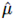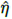U.S. Department of Transportation
Federal Highway Administration
1200 New Jersey Avenue, SE
Washington, DC 20590
202-366-4000

Federal Highway Administration Research and Technology
Coordinating, Developing, and Delivering Highway Transportation InnovationsThis report is an archived publication and may contain dated technical, contact, and link information
 Federal Highway Administration > Publications > Research Publications > 10065 > Modeling of Hot-Mix Asphalt Compaction: A Thermodynamics-Based Compressible Viscoelastic Model
 Publication Number: FHWA-HRT-10-065 Date: December 2010

# Modeling of Hot-Mix Asphalt Compaction: A Thermodynamics-Based Compressible Viscoelastic Model

## CHAPTER 4. NUMERICAL IMPLEMENTATION IN THE FE METHOD

The modeling framework and the proposed model used for the compaction study were introduced in chapter 3. The model was further developed and improved to account for the behavior of asphalt concrete subjected to compaction in the field. To this end, the model was implemented in the FE software CAPA-3D, developed at Delft University of Technology.(53,54) CAPA-3D was developed as an FE-based platform to serve the computational needs of the research group Mechanics of Structural Systems at Delft University of Technology and international teams that cooperate with the group. Over the years, the software has evolved into a full-fledged FE system for static or dynamic analysis of large-scale three-dimensional pavement and soil engineering models. The software consists of a sophisticated user interface; a powerful, highly parallelized band-optimizing mesh generator; a high-quality, user-controlled graphical output; several material and element types; and a variety of specialized algorithms for more efficient analysis of pavement construction. These algorithms include a moving load-simulation algorithm and a contact algorithm, which are essential for modeling the compaction process.

### VERIFICATION OF FE IMPLEMENTATION

Researchers solved the constrained compression problems using the FE implementation of the model in CAPA-3D. In finite elements, these problems were solved by employing a 20-noded three-dimensional solid element. The representative material element was constrained to move only in a normal direction (z-direction). A comparison was drawn between the solutions obtained from the analysis (with aid from calculations in MATLAB®) and the solutions obtained using the method of finite elements.(54) The compressive strain applied was 0.05, or 5 percent. The material properties used are presented in table 1. An explanation of the parameters listed in table 1 is presented in chapter 5 of this report. These parameters were also used for FE calculations.

Table 1. Parameters employed for the study of the material response when subject to one-dimensional constrained compression.(MPa)

n1

λ1

q1(MPa·s)

n2

λ2

q2

810

4.0

0.25

−15

1,400

2.5

0.25

−25

Inputs to the model are given by linearly increasing the input to a constant value and then keeping the input at this value. Removing the input is then performed by linearly decreasing from the maximum value to no input. The comparison for constant applied stress is presented in figure 24 and figure 25, and the comparison for constant applied strain is presented in figure 26 and figure 27. The calculated values (using MATLAB®) of the stretch and stress in response to applied stress and strain, respectively, and the corresponding FE solutions agree well, as can be observed from figure 24 through figure 27. This agreement serves as validation for the implementation of the model in CAPA-3D using the method of finite elements.

Figure 24. Chart. FE solution to the model response to applied constant stress—creep load.

Figure 25. Chart. FE solution to the model response to applied constant stress—total stretch.

Figure 26. Chart. FE solution to the model response to applied constant strain (compressive 0.05)—applied strain.

Figure 27. Chart. FE solution to the model response to applied constant strain (compressive 0.05)—normal stress response.

### CONSTANT SHEAR

In the developed FE implementation, a constant-shear problem is solved. The schematic representation for such a model is given in figure 28. The unit cube is subject to a rate-type shear deformation, and the boundary conditions to be applied to the unit cube are such that the cube is constrained to move only in a lateral direction, indicating a shear deformation.

Figure 28. Illustration. Schematic of the constant shear loading applied to a unit cube.

In figure 29 through figure 31, T12 represents the shear in the xy-plane, T11T22 represents the first normal stress, and T22T33 represents the second normal stress. As shown in the figures, the material response corresponds with that of a nonlinear material due to the exhibition of normal stress differences.

Figure 29. Chart. Shear stress (T12) observed in response to constant shear loading.

Figure 30. Chart. Comparison of the first normal stress (T11T22) response to constant shear loading.

Figure 31. Chart. Comparison of the second normal stress (T22T33) response to constant shear loading.

### CONSTANT SHEAR RATE

Using the developed FE implementation, a constant-shear-rate problem is solved. The schematic representation for such a model is given in figure 32. The unit cube is subject to a rate-type shear deformation, and the boundary conditions applied to the unit cube are such that the cube is constrained to move only in a lateral direction, indicating a shear deformation.

Figure 32. Illustration. Schematic of the constant shear rate applied to a unit cube.

In figure 33 through figure 36, T12 represents the shear in the xy-plane, T11–T22 represents the first normal stress, and T22–T33 represents the second normal stress.

Figure 33. Chart. Shear stress (T12) observed in response to constant shear rate loading.

Figure 34. Chart. Shear stress (T12) as a function of the shear rate (using the model parameters in table 1).

Figure 35. Chart. Comparison of the first normal stress (T11T22) response to constant shear rate loading.

Figure 36. Chart. Comparison of the second normal stress (T22T33) response to constant shear rate loading.

As shown in figure 33, figure 35, and figure 36, the material response corresponds with that of a nonlinear material due to the exhibition of normal stress differences (though small in comparison to the shear stress T12). For the chosen set of parameters, the model seems to exhibit near-linear shear-stress (T12) versus shear-rate characteristics. Such characteristics typically represent the response of a fluid with a rate of dissipation similar to Newtonian fluid. However, the model does exhibit normal stress differences, a typical characteristic of non-Newtonian fluids, as shown in figure 35 and figure 36. Hence, the model behaves like a non-Newtonian fluid, and the choice of the parameters enhances the shear-thickening nature of the model (unlike in figure 34). This feature of the model is very useful for the purposes of modeling compaction in the laboratory or in the field as the material in those situations undergoes shear-thickening behavior. The choice of model functions for shear modulus and viscosity ensure such a transition in the material's property.

 Return to top
Federal Highway Administration | 1200 New Jersey Avenue, SE | Washington, DC 20590 | 202-366-4000
Turner-Fairbank Highway Research Center | 6300 Georgetown Pike | McLean, VA | 22101